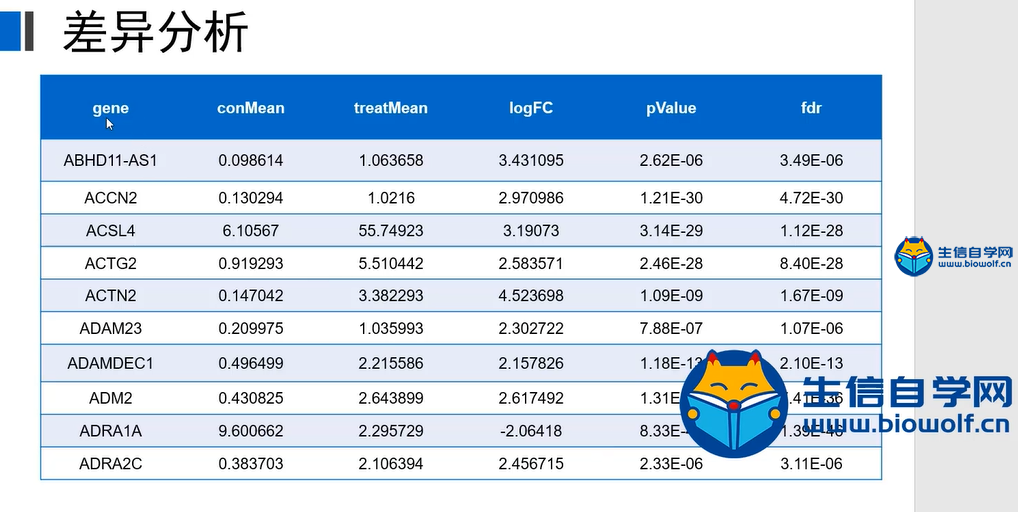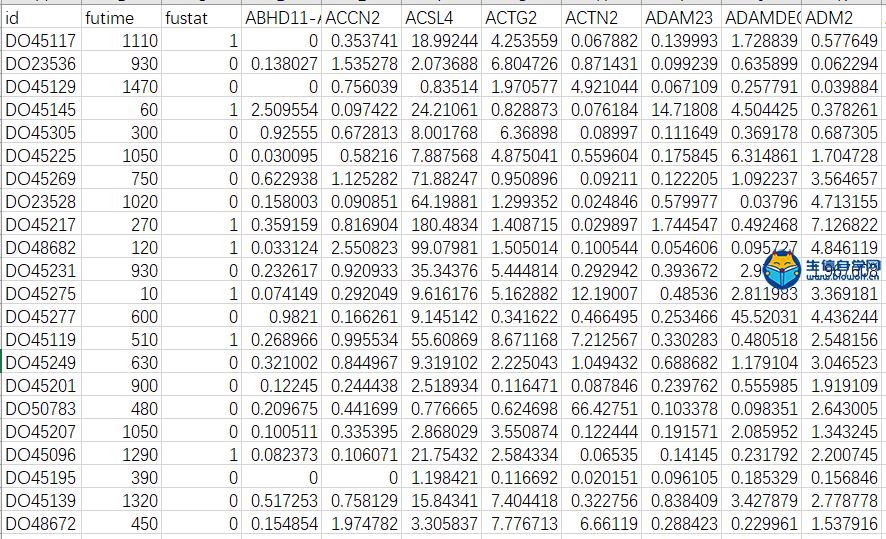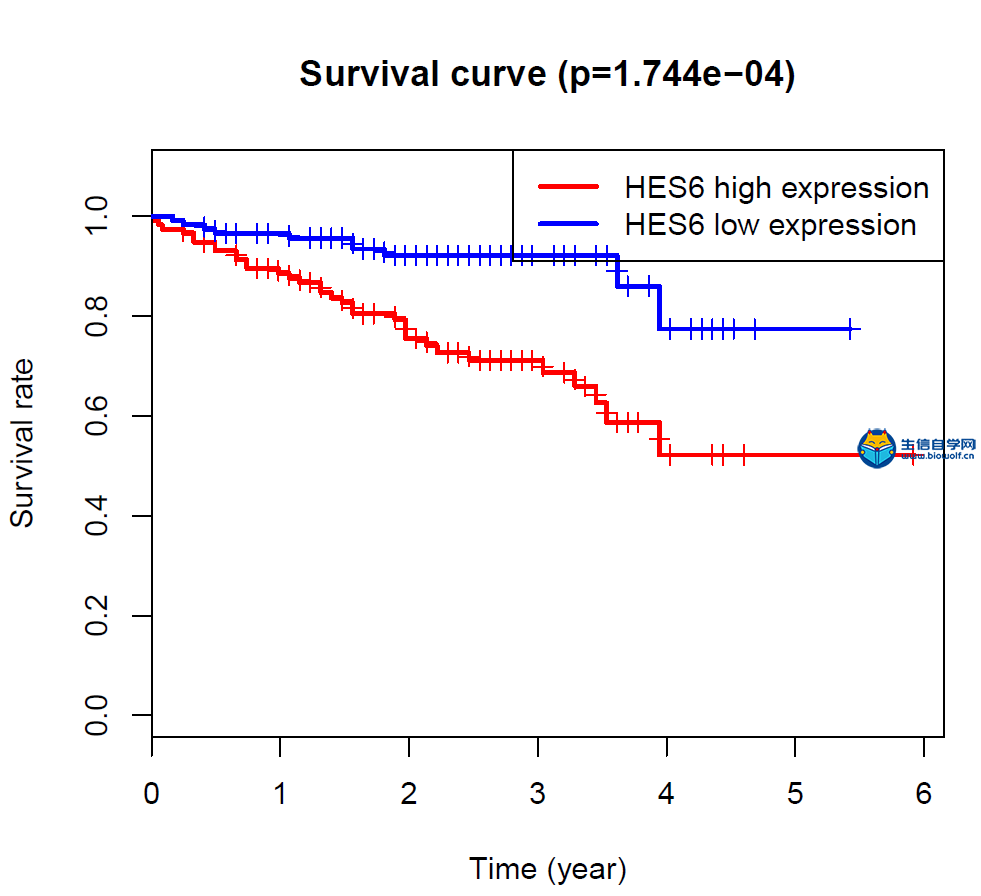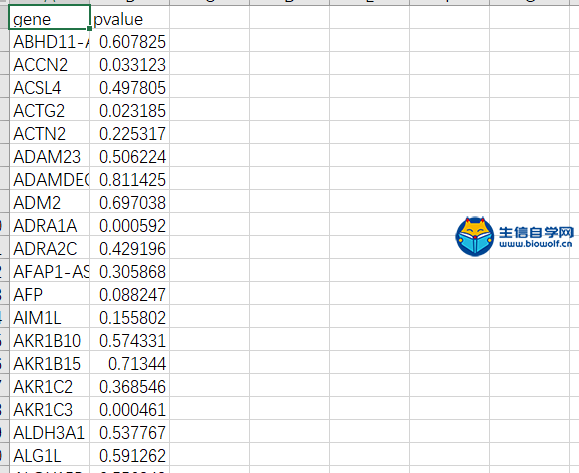### 差异分析生存分析热图绘制基于ICGC数据库1、下面我们来看下做差异的代码：

 ```if (!requireNamespace("BiocManager", quietly = TRUE)) install.packages("BiocManager") BiocManager::install("limma", version = "3.8")```

 ```library("limma") setwd("C:\\Users\\lexb4\\Desktop\\ICGCexp\\04.diff") #设置工作目录 inputFile="sampleExp.txt" #输入文件 fdrFilter=0.05 #fdr临界值 logFCfilter=2 #logFC临界值 conNum=202 #normal组样品数目 treatNum=240 #tumor组样品数目 #读取输入文件 outTab=data.frame() grade=c(rep(1,conNum),rep(2,treatNum)) rt=read.table(inputFile,sep="\t",header=T,check.names=F) rt=as.matrix(rt) rownames(rt)=rt[,1] exp=rt[,2:ncol(rt)] dimnames=list(rownames(exp),colnames(exp)) data=matrix(as.numeric(as.matrix(exp)),nrow=nrow(exp),dimnames=dimnames) data=avereps(data) data=data[rowMeans(data)>0.5,] 更多原创代码请联系生信自学网微信：18520221056 关注微信公众号“biowolf_cn"```2、接下来可以绘制一下热图，热图用到的输入文件就是我们做差异得到的diffGeneExp.txt，用到的包是pheatmap：

 ```install.packages("pheatmap") setwd("C:\\Users\\lexb4\\Desktop\\ICGCexp\\05.pheatmap") #设置工作目录 rt=read.table("diffGeneExp.txt",sep="\t",header=T,row.names=1,check.names=F) rt=log2(rt+0.001) library(pheatmap) Type=c(rep("con",202),rep("treat",243)) #修改对照和处理组样品数目 names(Type)=colnames(rt) Type=as.data.frame(Type) 获取生信自学网原创代码，可以直接购买课程```3、生存分析```install.packages("survival") setwd("C:\\Users\\lexb4\\Desktop\\ICGCexp\\07.survival") #工作目录（需修改） library(survival) pFilter=0.05 #由于图形太多，只对p小于0.05的基因绘图 rt=read.table("survivalInput.txt",header=T,sep="\t",check.names=F) #读取输入文件 rt\$futime=rt\$futime/365 #如果以月为单位，除以30；以年为单位，除以365 outTab=data.frame() ```BioWolf二维码生成器

(1)
100%

(0)
0%
------分隔线----------------------------